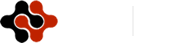International Journal of Energy Science and Engineering, Vol. 1, No. 3, July 2015 Publish Date: Jun. 24, 2015 Pages: 106-114

Numerical Investigation of Double Stack Blade Savonius Wind Turbine

Jaber Hosseinzadeh Gervi*, Mojtaba Aghajani Delavar, Korosh Sedighi

Department of Mechanical Engineering Babol Noshirvani, University of Technology, Babol, Iran

Abstract

Generating energy from wind as a renewable energy resource has always been a growing field of study. This article analyzes the aerodynamics of Savonius wind turbine with vertical axis. Blades with multi stacks can give rise to blade efficiency. For this purpose, different models of blades were suggested and the impact of blade intervals on the torque output has been studied. Moreover, the flow of fluid around the turbine rotor has been calculated with the CFD method and the standard k-ε turbulence model is used. The simulations were performed at four different wind velocities of 3, 4, 5, and 6 m/s. The rotor torque is analyzed with different rotor angles from 0 to 360 degrees with 30° pitches. The results clearly show that the torque output increases as the intervals between the blade stacks decrease. Also this paper, for the first time, shows that as the thickness of the outer blade’s slot increases, the torque will decrease.

Keywords

Savonius Rotor, Computational Fluid Dynamics, Double Stacks Blade, Torque

1. Introduction

In this model the blade is designed such that the rotor incident flow between the inner and outer blade is contracted and generates extra torque.

2. The Studied Geometry and Boundary Conditions

The studied geometry is originally designed from the proposed one by Mactavish et al. . Their model presents double stacks blade. The outer blade is a semi cylinder with diameter of 127 mm and a slot with the thickness of d is inserted in the middle of it. This slot has two functions; first it avoids the negative torque caused by the clash of recursive flow from the outer blade to the inner one. Second; since negative pressure exist behind the blade, the flow sinks into the slot and induces an extra torque. The diameter of the inner blade and the thickness of each blade are set to be 50 mm and 2 mm, respectively. The outer blade’s slot thickness and the intervals between blades (h) are the factors that have influence on the rotor’s torque output.

The torque output was simulated and compared for different cases. For the angles between 0 to 360° with the 30° step, analysis of rotor’s torque is performed. In order to calculate the torque output in this simulation, the thickness of the slot was set to be d=7 and d=14 mm. Moreover, different thickness of h=4, 14, and 24 at velocities of 3, 4, 5, and 6 m/s are simulated.

3. Numerical Method

In this paper FLUENT 6.3 has been used for simulations. As fig 1 shows, in this method the control volume is divided in two sub domain. Domain one includes the rotor and its around which has a constant rotational speed which is proportional to the incident wind velocity and blade tip speed ratio. Hence, the second domain is without the rotational speed and just contains the input wind velocity.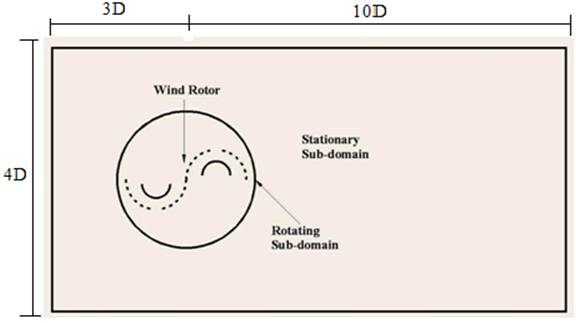Fig. 1. Overall computational domain of the VAWT.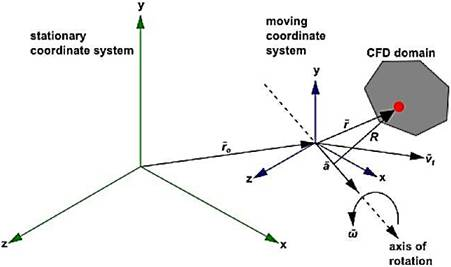Fig. 2. Stationary and Moving Reference Frame .

Also, for rotor’s rotation simulation in steady state the Moving References Frame (MRF) method is used. SIMPLE algorithm was used for calculating the velocity field along with pressure.

4. The Governing Equations

The mathematical equations can be defined as a composition of dependent and independent variables and relative parameters in the form of differential equations which govern physical phenomena. In the following sections some differential equations that rule here are presented.

4.1. The Continuity Equation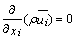Eq. (1)

Whereis density and V is velocity vector.

4.2. The Momentum Equation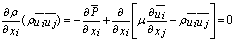Eq. (2)

In whichis density and V is velocity vector and p,,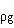, and F are the static pressure, stress tensor, gravitational force of the object and external force, respectively. The viscous stress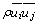given by equation (2), is calculated as following: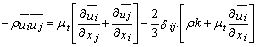Eq. (3)

The transport equations considerate for the turbulences quantities from RNG k-ε model are described by Equations (4) and (5).

4.3. The turbulence Model

In this paper the k-ε standard model is used which can be demonstrated as: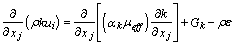Eq. (4)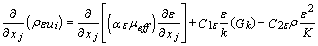Eq. (5)

In these equations,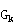represents the generation of turbulent kinetic energy due to the mean velocity gradients, and the quantities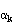and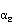are the inverse effective Prandtl numbers for k and ε, respectively. The effective Viscosity,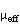, is calculated by the combination of the turbulent kinetic energy (k ) and the dissipation ratio (ε).

5. Validation and Grid Check

Meshing was performed with Gambit software. The number of elements for the two dimensional calculation are 17000.The Validation was done with the paper of Hadi Ali  which had done an experimental research in wind tunnel on a two blades rotor with 200 mm diameter at 5.3 m/s wind speed. Fig 3 shows the geometry of the documentation.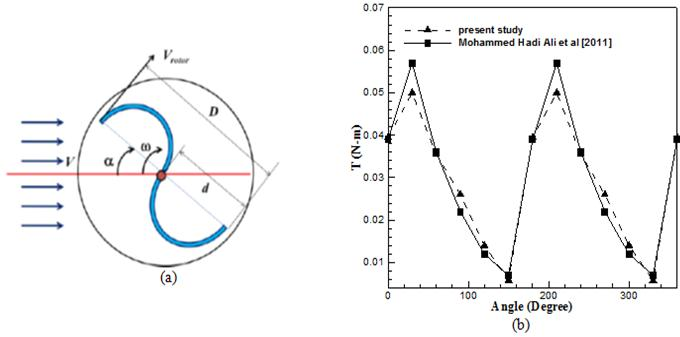Fig. 3. a) Geometry of validation , b) Validation of the steady numerical predictions with experimental torque results.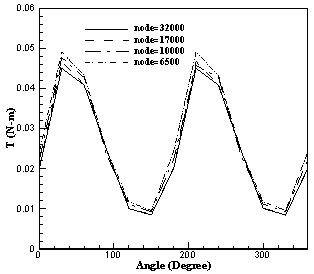Fig. 4. Independent of mesh.

As fig 4, shows the increasing of mesh numbers just influence the result at 30 and 60 degree and finer mesh results in more exact output for these angles. As it can be seen in this figure, mesh independency happens at mesh number of 17000, that is why it is chosen for simulations.

6. Results and Discussion

As discussed in previous sections, the torque output is influenced by two factors. The simulation is performed for the mentioned geometry at different cases and the torque output is calculated for each case at different velocities. An analogy between two rotors with simple blade and double stacks blade at 5 m/s is illustrated in fig 5. Fig 5 shows that,  due to the increasing contraction area between the blades and flow, and also contraction of the flow between the two blades, pressure increases and as a result the torque increases, too.

The torque output for a rotor with double stacks blade is more that the simple blade one. The existence of an inner blade in the rotor plays an important role here. It can be easily seen in fig 6 that the less this inner blade barricades the flow, the less the torque output become. It means that torque of the proposed model surpass the simple rotor at the zero degree and maximize at 30°. The reason is that the inner blade is located completely in front of the flow at 30° and its aerodynamics condition is suitable. Furthermore, at 60° the torque decreases but unlike the simple rotor model, it is more than the torque at zero degree. Again the inner blade caused the torque output at 90° to surpass its amount at zero degree. Although by shifting the angle to 120° the inner blade’s effects decreases, but still its performance is superior to that of the rotor with simple blade. This reduction in torque continues until 150o at which the performance of the new model is similar to the simple model. The pressure contours at 5 m/s for 30° for all cases are shown in fig 7.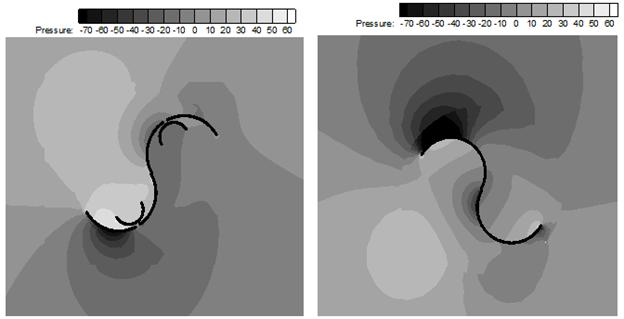Fig. 5. A Comparison between rotor’s pressure contour for simple blade and double stacks blade at the rotor Rotation angle of 30 degree.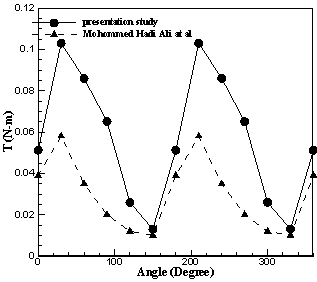Fig. 6. A Comparison with rotor torque for two blade and proposed models.

Fig 8 shows that as the wind velocity get higher, the rotor’s torque increases, too. According to the above description, the rotor’s torque does not change so much at the angle of 120 and 150°. The reason of torque decrement can be seen obviously in fig 8. The inner blade has its maximum efficacy with rotor rotation at 30° (fig 9). When rotor reaches 120°, this blade’s efficacy decreases and experiences its minimum at 150°.

The thickness of the slot located at the outer blade’s back is another factor that has great influence on the torque output of the rotor. Fig 10 shows an analogy between the slot thickness of 7, 14, and 21 mm with the constant stacks interval of 24 mm and at the speed of 6 m/s.

As can be clearly seen in fig 10 the larger the  outer blade slot thickness, the lower the torque output. Because the slot thickness is greater the input wind flow easily back removed and a little momentum is built.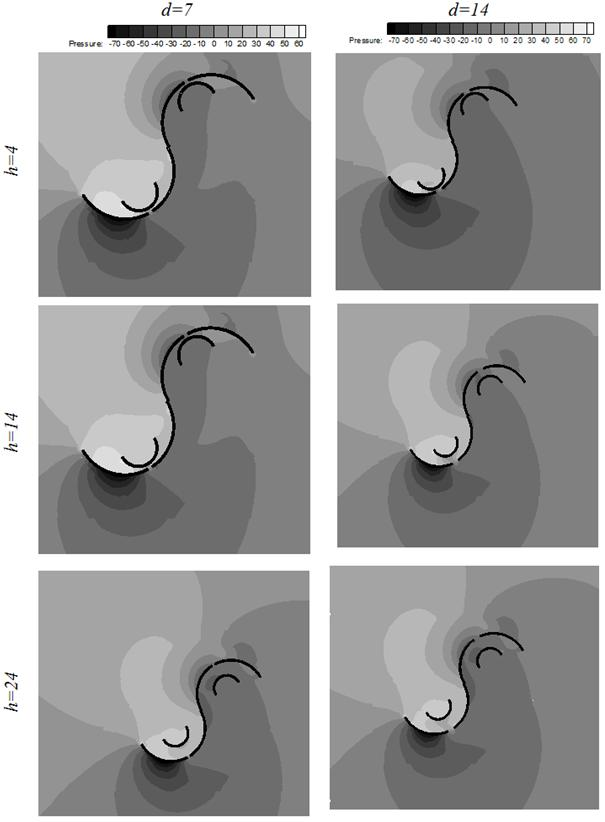Fig. 7. Contour of pressure for a 30 ° angle and speed 5m/s for all modes.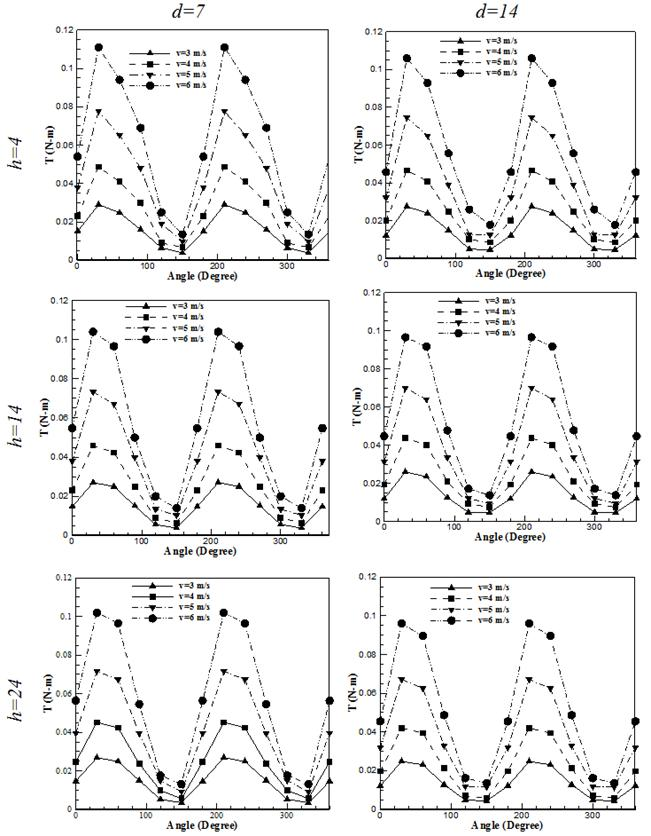Fig. 8. Torque at different speeds in different d and h.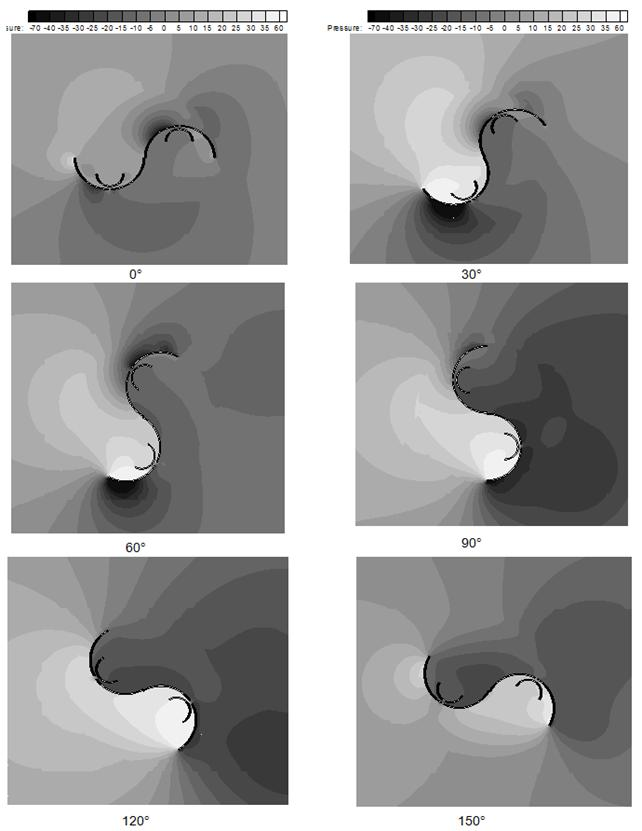Fig. 9. Contour of pressure on the blade away from 0 to 150 degrees in steps of 30° in d=4mm and h= 7mm.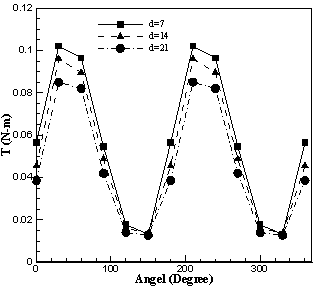Fig. 10. Comparison of output torque in thickness of 7, 14 and 21 mm.

7. Conclusion

This article, for the first time, studies the aerodynamics performance of vertical axis Savonius wind turbine with double stacks blades rotor. The following results are drawn:

·     The mean value of torque output of a rotor with double stacks blade is more than a rotor with simple blade

·     The closer the stacks blade to the main blade, the more rotor torque output

·     For larger main blade thickness, the fluid easily flows around the blades and as a result the torque output decreases

·     As one might expected when the input flow velocity increases, the rotor torque output also increases

References

1. Sukanta Roy, Ujjwal K. Saha, " Review on the numerical investigations into the design and development of Savonius wind rotors", Renewable and Sustainable Energy Reviews, VOL.24, pp. 73-81, 2013
2. S.J. Savonius. The S-rotor and its applications. J. Mech. Engg. 53, 333- (1931338).
3. Khandakar Niaz Morshed, Mosfequr Rahman, Gustavo Molina, Mahbub Ahmed. Wind tunnel testing and numerical simulation on aerodynamic performance of a three-bladed Savonius wind turbine. International Journal of Energy and Environmental Engineering. 2013, 4:18
4. Mohommed Hadi Ali. Experimental Comparison Study for Savonius Wind Turbine of Two & Three Blades At Low Wind Speed. International Journal of Modern Engineering Research (IJMER) .Vol. 3, Issue. 5, Sep - Oct. 2013 pp-2978-2986
5. M.H. Nasef, W.A.El-Askary, A.A.AbdEL-hamid, H.E.Gad. Evaluation of Savonius rotor performance: Static and dynamic studies. J. Wind Eng.Ind.Aerodyn.123(2013)1–11
6. Pinku Debnath, Rajat Gupta. Flow Physics Analysis of Three-Bucket Helical Savonius Rotor at 90 ᵒTwist Angle Using CFD. International Journal of Modern Engineering Research (IJMER). Vol.3, Issue.2, March-April. 2013, pp-739-746
7. Bachu Deb and, Rajat Gupta. Fluid Flow Analysis of Savonius Rotor at Different Rotor Angle Using CFD. ISESCO JOURNAL of Science and Technology, Vol .8 - No2012 ( 35-42)
8. S. McTavish, D. Feszty, T. Sankar. Steady and rotating computational fluid dynamics simulations of a novel vertical axis wind turbine for small-scale power generation. Renewable Energy. 41. (2012). 171-179
9. M.H. Nasef, W.A. El-Askary, A.A. AbdEL-hamid, H.E. Gad, "Evaluation of Savonius rotor performance:Static and dynamic studies", Journal of Wind Engineering and Industrial Aerodynamics, pp.1-11, 2013
10. Fluent Inc Products, FLUENT® Ver. 6.3.26

 Contents 1. 2. 3. 4. 4.1. 4.2. 4.3. 5. 6. 7.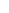600 ATLANTIC AVE, BOSTON,
MA 02210, USA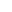+001-6179630233
JournalsJournal ListsAuthor GuidelinesPayment InformationSubmit a ManuscriptOpen Special IssuesJoin as Editorial MembersJoin as ReviewersPrivacy NoticeTerms of ConditionsHelp Center